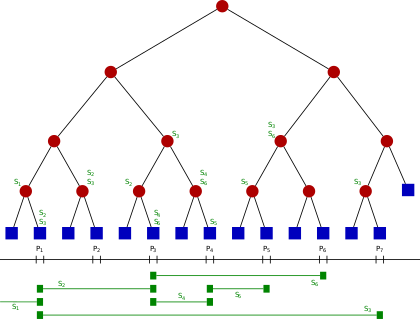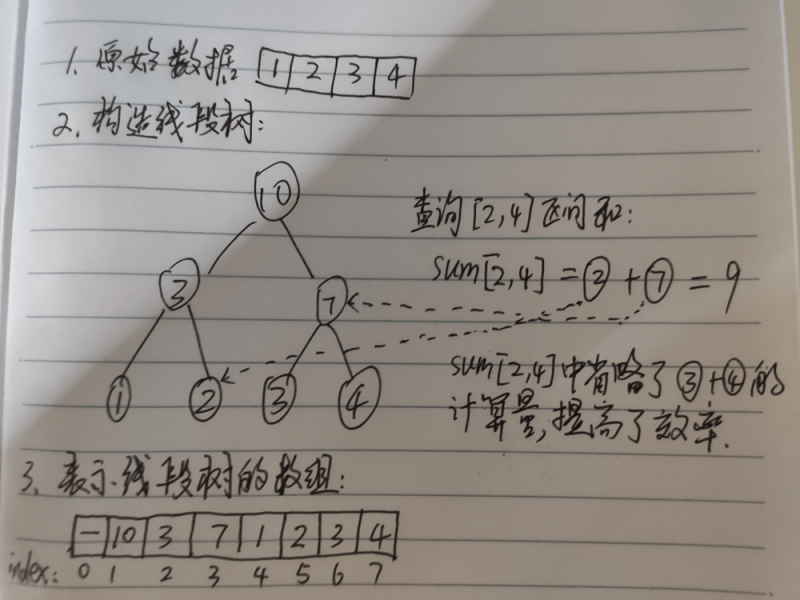# 线段树（Segment Tree）zayfen﻿

﻿

﻿

﻿

1. 求区间和

2. 求区间的最小值

3. 求区间的最大值

﻿

## 三、线段树的原理

﻿

﻿

﻿

﻿

﻿

﻿﻿

## 四、线段树的区间求和实现

﻿

rust的实现（区间求和）：

﻿

#[derive(Debug, Clone)]pub(crate) struct NumArray {  tree: Vec<i32>}// i的左孩子，因为数组从index=1开始，// 所以，left_child是i*2, 而不是 i*2+1pub(crate) fn left_child (i: usize) -> usize {  i << 1}// i节点的右孩子，因为数组从index=1开始，// 所以，right_child是i*2+1, 此处为了提高效率，用(i<<1) | 0b1来表示i*2+1// 以为 i<<1 最低位一定是0, 所以 ((i<<1) | 0b1)使最低位变为为1, 就表示加1pub(crate) fn right_child (i: usize) -> usize {  (i << 1) | 0b1}// i的父节点pub(crate) fn parent (i: usize) -> usize {  i >> 1 as usize}impl NumArray {    // 构造线段树  fn new (nums: Vec<i32>) -> Self {    let mut tree_arr = vec![];    let len = nums.len();    tree_arr.resize(len, 0);      // 构造线段树的数组长度是原数据数组(nums)的长度2倍      // 将原始数据放在线段数组的尾部，使其成为叶子节点      // 线段数组从索引1开始，所以最后的节点的父节点就是 last_index / 2, and last_index = (tree_arr.len() - 1)    let mut tree_arr = [tree_arr, nums].concat();    // (1..5).rev() => 4 3 2 1    for i in (1..len).rev() {      // now i from len-1 to 0 => [len-1, 0)      dbg!(i);      tree_arr[i] = tree_arr[left_child(i)] + tree_arr[right_child(i)];    }	      // 数组构造完成    NumArray {      tree: tree_arr    }  }    // 更新线段树节点，同时要更新父节点  fn update (&mut self, i: usize, val: i32) {    let n = self.tree.len() / 2;    // i must between [0, n)    if i >= n {      panic!(format!("{} is out of bounds", i));    }    let index = n + i;    self.tree[index] = val;    // update parent    let mut p = parent(index);    // because self.tree's index is from 1    while p != 0 {      self.tree[p] = self.tree[left_child(p)] + self.tree[right_child(p)];      p = parent(p);    }  }  // 计算和 [i, j), 左开右闭区间  fn sum_range (&self, i: usize, j: usize) -> i32 {    let mut res = 0;    let n = self.tree.len() / 2;    let mut left = n + i;    let mut right = n + j;    while left < right {              // 当左节点属于父节点的右子节点时，不能使用父节点的区间值，      // 所以，需要单独计算，计算完成之后，需要从左边缩小求和区间，      // 因此left += 1, e.g.：sum[3, 7） => sum[3,4) + sum[4, 7)      if left & 0b1 == 1 { // 判断节点是右子节点，因为右子节点的最低位是1，见right_child()函数        res += self.tree[left];        left += 1;      }        	  // right是右子节点，因为是开区间，只有左子节点要计算，因为粗不能使用父节点，所以需要单独加上左子节点值      if right & 0b1 == 1 { // 判断节点是右子节点        right -= 1;        res += self.tree[right];      }		      // 因为left指向左子节点，所以可以使用父节点的区间值      // 因为right指向左子节点，right是开区间，      // 所以right指向的左子节点右边的需要计算在内，所以right可以指向其父节点      left >>= 1;      right >>= 1;    }    res  }}// 测试#[cfg(test)]mod range_sum_query_tests {  use super::*;  #[test]  fn test_num_array () {    let arr = NumArray::new(vec![1, 2, 3, 4, 5]);    let ref_arr = &arr;    dbg!(ref_arr);    let sum = arr.sum_range(0, 4);    dbg!(sum);    assert_eq!(10, sum);    let mut arr = arr;    arr.update(0, 2);    let sum = arr.sum_range(0, 4);    assert_eq!(11, sum);  }}

﻿## 评论